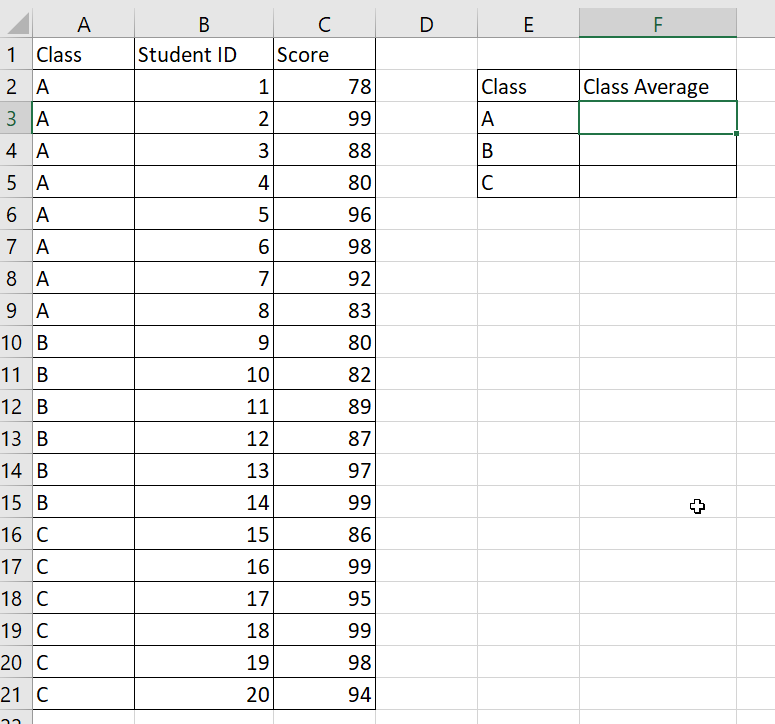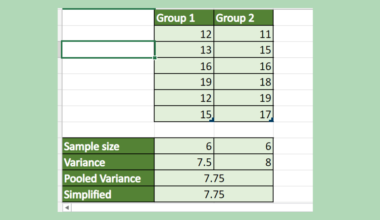# How To Calculate Conditional Mean in Excel

This article will explain how to calculate the conditional mean in Excel.

We can use the AVERAGEIF function to find the conditional mean given a range of values.

Excel users often use the `AVERAGE` function to find the mean of a given range of values. However, there may be cases where you want to selectively choose what values to take when finding the mean.

For example, you may have a dataset of student scores from various classes. We want to compare the average of one class with another to see if there is a significant difference.

We can use the `AVERAGEIF` and `AVERAGEIFS` functions to find this conditional mean in Excel. The functions require users to specify at least two ranges: the range to compare with the criteria, and the actual numerical range we want to find the average of.

Using the `AVERAGEIF` function enables us to find the mean of a certain subset of a dataset without using a filter or any other function.

Now that we know when to calculate the conditional mean, let’s learn how to apply it to a sample spreadsheet.

## A Real Example of Calculating the Conditional Mean in Excel

The following section provides a few examples of how to calculate conditional means in Excel. We will also explain the formulas and tools used in these examples.

First, let’s take a look at our sample given. We have a dataset of student scores with their associated class and student ID. We want to compare students’ average scores in Class A, B, and C.The `AVERAGEIF` function can help create a new table that finds students’ average scores in each class. After using the formula, we determined that Class C had the highest average score.To get the values in Column F, we just need to use the following formula:

`=AVERAGEIF(\$A\$2:\$A\$21;E3;\$C\$2:\$C\$21)`

The formula above uses the values in Column A to determine whether or not a particular score is included in the mean calculation. For example, the formula in cell F3 will only include values in Column C where the class in Column A is equal to E5 or “A”.

We can also use the `AVERAGEIFS` function to find the conditional mean if we require more than one criteria.In the example above, we need to check that the following criteria is met: the student belongs to class A, and the student has an age of 16 or older.

Do you want to take a closer look at our examples? You can make your own copy of the spreadsheet above using the link attached below.

Use our sample spreadsheet to see how we calculated the conditional mean from our sample dataset.

If you’re ready to try calculating the conditional mean in Excel, head over to the next section to read our step-by-step breakdown on how to do it!

## How to Calculate the Conditional Mean in Excel

This section will guide you through each step needed to calculate the conditional mean in Excel. We’ll explain how to use the `AVERAGEIF` function for both categorical and numerical conditions. We will also briefly explain how to work with multiple criteria with the `AVERAGEIFS` function

Follow these steps to begin calculating the conditional mean in Excel:

1. First, we’ll create a new table to place the results of our `AVERAGEIF` function.2. Select an empty cell and type the formula “=AVERAGEIF(“ to start using the `AVERAGEIF` function.3. Type the range that we want to compare with our criteria as the first argument of the `AVERAGEIF` function.In this example, we’ve selected the range
A2:A21 since we’re concerned with what class the score comes from.
4. Next, type the criteria itself as the second argument.Since we are only interested in values where the class is equal to “A”, we can just type a reference to cell
E4.
5. Next, we’ll need to type the cell reference of the range we want to compute the mean of.In this example, we’ve selected the range
C2:C21 since these are the scores that we want to find the conditional mean of.
Do note that the size of the first and third arguments must be equal for the `AVERAGEIF` formula to work.
6. Hit the Enter key to evaluate the function.In the example above, we’ve determined that the average score in Class A is 89.25. If we compute the mean manually with the `AVERAGE` function, we should achieve the same result.
7. To find the conditional mean of the other classes, we must convert the first and third arguments into absolute references.Afterward, the user can use the AutoFill tool to drag the formula down.
8. We can also use the `AVERAGEIF` function with numerical conditions. For example, we can choose to find the average score for students aged 16 and older.9. If you need to check for more than one condition, we can use the `AVERAGEIFS` function instead.10. The first argument of `AVERAGEIFS` will be the range with the values we want to find the mean of.11. The next two arguments will indicate the first criteria to check.12. Users can continue to add more pairs of arguments to specify additional criteria to consider when finding the conditional mean.These are all the steps needed to calculate the conditional mean in Excel.

This detailed guide should provide you with all the steps you need to start calculating the conditional mean in Excel.

We’ve explained how to use the `AVERAGEIF` and `AVERAGEIFS` functions to find the mean of a range of values based on certain criteria.

The `AVERAGEIF` function is just one example of the many Excel functions you can use in your spreadsheets. Our website offers hundreds of other functions and methods to help you get more out of Microsoft Excel.

With so many other Excel functions available, you can find one appropriate for your use case.

Don’t miss out on our team’s new spreadsheet tips, tricks, and best practices. Subscribe to our newsletter to stay updated on the latest guides from us!### Get emails from us about Excel.

Our goal this year is to create lots of rich, bite-sized tutorials for Excel users like you. If you liked this one, you'd love what we are working on! Readers receive ✨ early access ✨ to new content.

##### You May Also Like## How to Calculate Pooled Variance in Excel

This guide will explain how to calculate pooled variance in Excel using the VAR.S function. The rules for…## How to Use Wildcard in SUMIFS Function in Excel

This guide will discuss how to use wildcards in the SUMIFS function in Excel.  The rules for using…## How to Perform Logistic Regression in Excel

This guide will explain how to perform logistic regression in Excel. We’ll explain how to use the maximum…## How to Create a Lorenz Curve in Excel

This guide will explain how to create a Lorenz curve in Excel. You’ll learn how to use the…